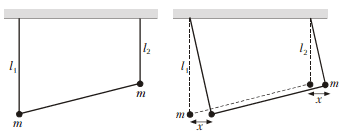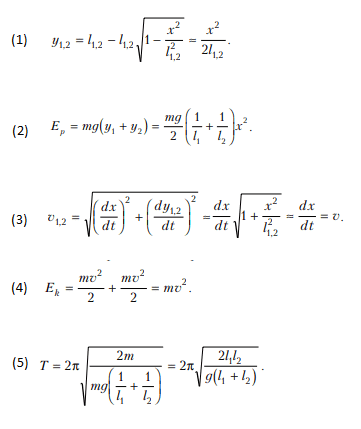# Determine the period of small oscillations

Jorgen1224

## Homework Statement

Two balls of mass m are attached to ends of two, weigthless metal rods (lengths l1 and l2). They are connected by another metal bar.
Determine period of small oscillations of the system## Homework Equations

Ek=mv2/2
v=dx/dt
Conversation of energy
2πsqrt(M/k)

## The Attempt at a Solution

That is actually the problem. I know the solution, but i don't really understand it and i wanted to ask if anyone could explain it to me or help me understand. I'm especially curious about the 1st and 3rd lines.

1st equation i don't understand at all, where did it come from? This root makes me think that pythagora's theorem was used, but how did it even turn into this fraction at the end?

I understand the beginning of 3rd equation, i suppose that's pythagoras theorem there, but why written in derivatives? It's basically vx and vy And how did this square root turn into a different one, but times dx/dt? Apparentaly this root is equal 1.#### Attachments

Mentor
You know that ##\cos(\theta) = \sqrt{1- \sin(\theta)^2}##. Consider the small angle approximation for your scenario.

Homework Helper
Gold Member
2022 Award
i don't really understand it
There is a small angle approximation in the diagram itself. In reality, the horizontal displacements will not be equal. The exact relationship is ##l_1l_2(1-\cos(\theta_1-\theta_2))=h(l_2\sin(\theta_2)-l_1\sin(\theta_1))##, where h is the horizontal distance between the string supports. But the LHS is order θ2, whereas the RHS is order θ, so to a first order approximation ##l_2\sin(\theta_2)=l_1\sin(\theta_1)##.
Further approximations in the algebra are just more of the same.

Handling approximations correctly can be a bit tricky. You might keep the first two order terms (a constant and θ term) and discard the rest (θ2 and up) only to find that the terms you kept all cancel later and you should have kept the quadratic term. Generally speaking, though, sin(θ) can be safely turned into θ (discarding θ3 and up) and cos(θ) into 1-½θ2.

At the end of eqn 3, there is a factor like √(1+x2). This can be expanded by the standard method as 1+½x2-x4/8... At this stage, it can be seen that the 1 is not going to cancel with anything, so we can safely discard the x2 term onwards.

•gneill
Jorgen1224
Okay, now i think i do understand 3rd equation, but I'm still stuck at the first one. In this equationl1l2(1-cos(θ12))=h(l2sin(θ2)-l1sin(θ1)) if we make an approximation l_2\sin(\theta_2)=l_1\sin(\theta_1) doesn't that make the right side equal to zero?

And should i use here l1l2(1-cos(θ12)) formula for cos(θ12)=cosθ1cosθ2+sinθ1sinθ2? If so, what then? I don't see it disappearing anyhow.

I'm sorry, this is my first time ever with such approximations and I'm getting lost in math here a little bit too.

Last edited:
Jorgen1224
And where did that relationship come from? i mean l1l2(1-cos(θ12))=h(l2sin(θ2)-l1sin(θ1))

Homework Helper
Gold Member
2022 Award
And where did that relationship come from? i mean l1l2(1-cos(θ12))=h(l2sin(θ2)-l1sin(θ1))
That's just geometry. To get it, express the length of rod connecting the masses in terms of the other rod lengths, the distance between the supports, and the angles the hanging rods make to the vertical. Equate it to that same length when the angles are zero.
But I only went through that exercise because it seemed to me that the assumption in the diagram that the horizontal displacements ("x") are near enough equal was not quite obvious.
So the choice for you is to accept the diagram as given or develop the equation I posted and make the approximation from there.

Homework Helper
Gold Member
2022 Award
In this equationl1l2(1-cos(θ1-θ2))=h(l2sin(θ2)-l1sin(θ1)) if we make an approximation l_2\sin(\theta_2)=l_1\sin(\theta_1) doesn't that make the right side equal to zero?
Yes, that's the point. As we make the two angles smaller and smaller, the left hand side gets smaller like θ2, whereas the sin θ terms on the right only get smaller like θ. So the difference between ##l_2\sin(\theta_2) ## and ##l_1\sin(\theta_1) ## must get smaller like θ2.

Staff Emeritus
Homework Helper
Gold Member
That's just geometry. To get it, express the length of rod connecting the masses in terms of the other rod lengths, the distance between the supports, and the angles the hanging rods make to the vertical.

As I read the problem, I interpret it as: the motion is entirely in the vertical plane,

Homework Helper
Gold Member
2022 Award
As I read the problem, I interpret it as: the motion is entirely in the vertical plane,
In the vertical plane containing the three rods, yes. What did I write that suggested otherwise?

Staff Emeritus
Homework Helper
Gold Member
In the vertical plane containing the three rods, yes. What did I write that suggested otherwise?
The following from Post #6 .
That's just geometry. To get it, express the length of rod connecting the masses in terms of the other rod lengths, the distance between the supports, and the angles the hanging rods make to the vertical. Equate it to that same length when the angles are zero.
Admittedly, I did not make an attempt to replicate your result with that or any other assumption.

A second look: My considering that you might think that the motion was perpendicular to the vertical plane (passing through the rod hangers) is rather silly. (DUH ! ) No way would ##x## be even close to the same for the two balls.

Jorgen1224
And I'm still extremely confused. I do understand now the beginning of the first equation, but I don't see where did x2/2l1,2 come from. How was this calculated?